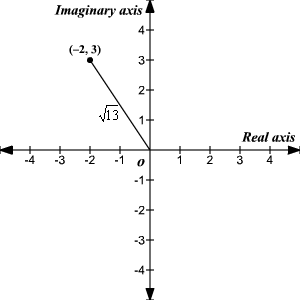# Absolute Value of a Complex Number

The absolute value of a complex number , $a+bi$ (also called the modulus ) is defined as the distance between the origin $\left(0,0\right)$ and the point $\left(a,b\right)$ in the complex plane.

$|\text{\hspace{0.17em}}a+bi\text{\hspace{0.17em}}|=\sqrt{{a}^{2}+{b}^{2}}$

Example:

$\begin{array}{l}|\text{\hspace{0.17em}}-2+3i\text{\hspace{0.17em}}|=\sqrt{{\left(-2\right)}^{2}+{3}^{2}}\\ \text{\hspace{0.17em}}\text{\hspace{0.17em}}\text{\hspace{0.17em}}\text{\hspace{0.17em}}\text{\hspace{0.17em}}\text{\hspace{0.17em}}\text{\hspace{0.17em}}\text{\hspace{0.17em}}\text{\hspace{0.17em}}\text{\hspace{0.17em}}\text{\hspace{0.17em}}\text{\hspace{0.17em}}\text{\hspace{0.17em}}\text{\hspace{0.17em}}\text{\hspace{0.17em}}\text{\hspace{0.17em}}\text{\hspace{0.17em}}\text{\hspace{0.17em}}=\sqrt{4+9}\\ \text{\hspace{0.17em}}\text{\hspace{0.17em}}\text{\hspace{0.17em}}\text{\hspace{0.17em}}\text{\hspace{0.17em}}\text{\hspace{0.17em}}\text{\hspace{0.17em}}\text{\hspace{0.17em}}\text{\hspace{0.17em}}\text{\hspace{0.17em}}\text{\hspace{0.17em}}\text{\hspace{0.17em}}\text{\hspace{0.17em}}\text{\hspace{0.17em}}\text{\hspace{0.17em}}\text{\hspace{0.17em}}\text{\hspace{0.17em}}\text{\hspace{0.17em}}=\sqrt{13}\end{array}$